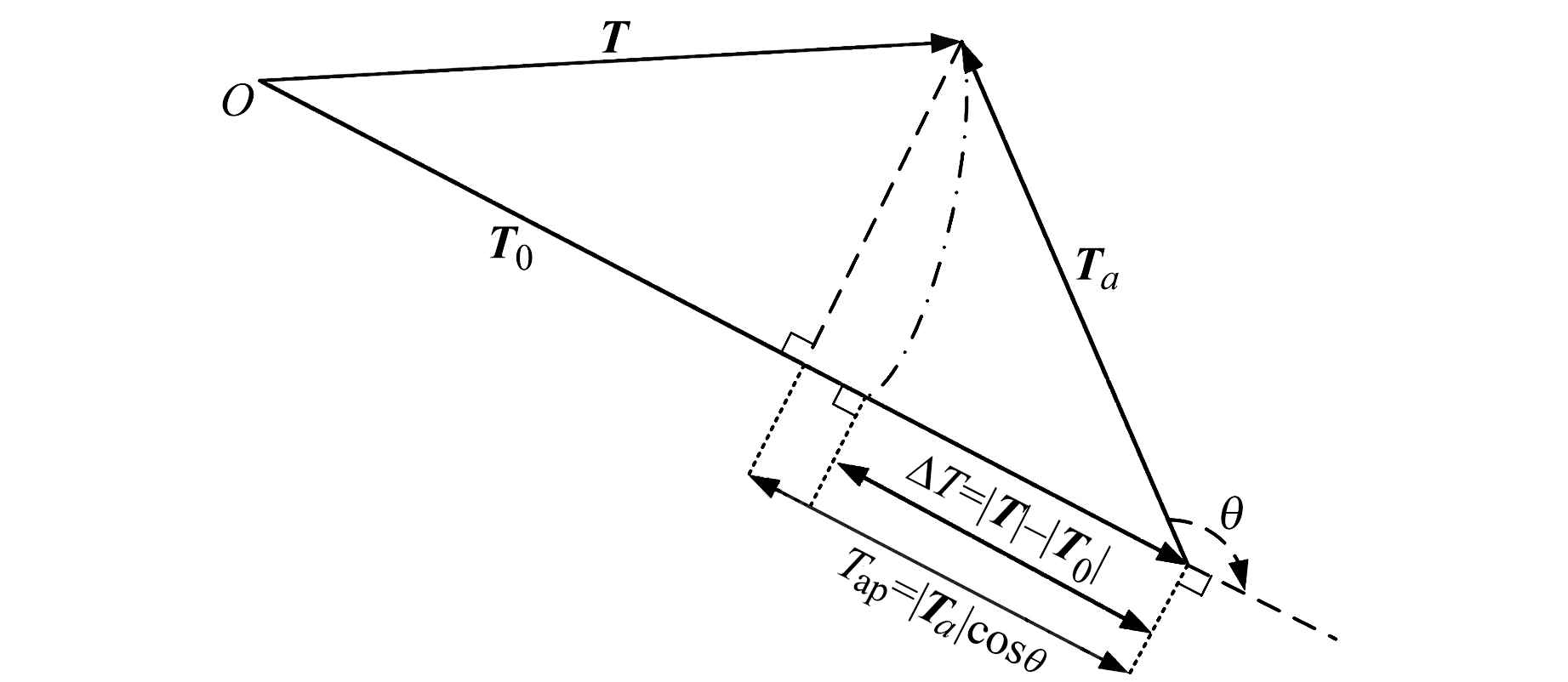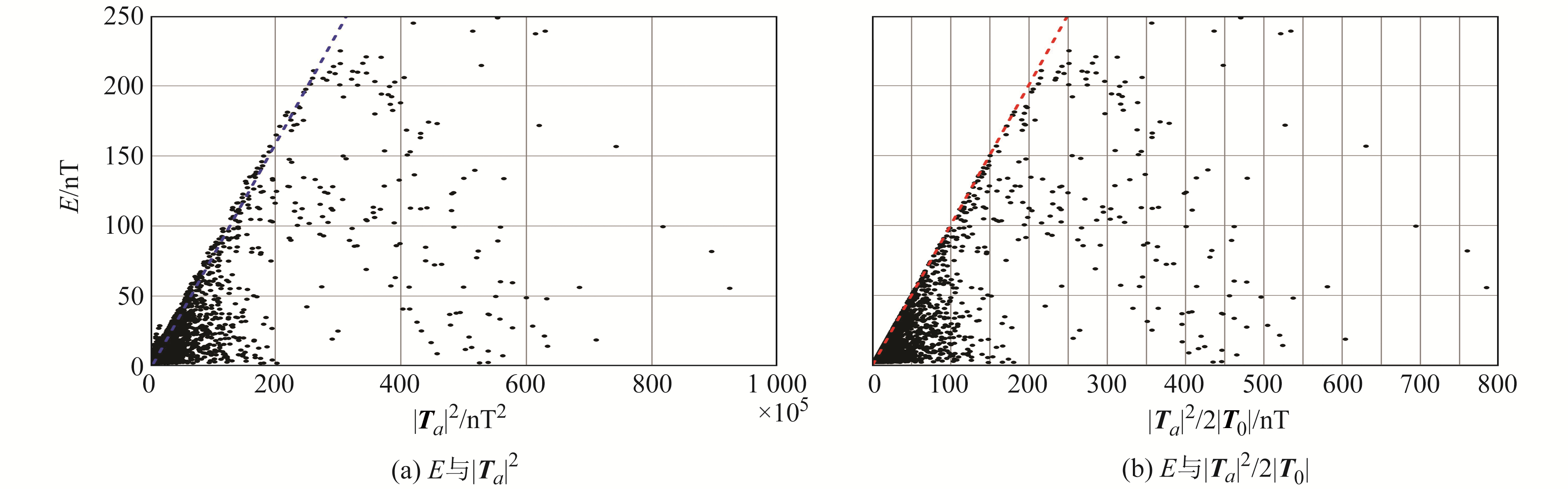﻿ 利用全球地磁场模型研究Δ<i>T</i>与<i>T</i><sub>ap</sub>之间的差异性特征文章快速检索 高级检索
 大地测量与地球动力学2021, Vol. 41Issue (11): 1141-1145, 1151  DOI: 10.14075/j.jgg.2021.11.008### 引用本文CHEN Kang, HU Zhengwang, DU Jinsong. Research on the Differential Characteristics between ΔT and Tap Using the Global Geomagnetic Field Model[J]. Journal of Geodesy and Geodynamics, 2021, 41(11): 1141-1145, 1151.### Foundation support

National Natural Science Foundation of China, No.41604060; Independent Research Project of the State Key Laboratory of Geological Processes and Mineral Resources, China University of Geosciences, No.MSFGPMR01-4.

### Corresponding author

HU Zhengwang, lecturer, majors in geophysical teaching and research, E-mail: hzw@cug.edu.cn.

### 第一作者简介

CHEN Kang, senior engineer, majors in geophysical exploration, E-mail: chenkang_gsi@163.com.

### 文章历史

1. 广西壮族自治区第七地质队，广西壮族自治区柳州市柳堡路3号，545100;
2. 中国地质大学(武汉)地球物理与空间信息学院地球内部多尺度成像湖北省重点实验室，武汉市鲁磨路388号，430074;
3. 中国地质大学(武汉)地质过程与矿产资源国家重点实验室，武汉市鲁磨路388号，430074

1 ΔTTap差异性的理论分析

 $\begin{gathered} \Delta T=|\boldsymbol{T}|-\left|\boldsymbol{T}_{0}\right| \end{gathered}$ (1)
 $T_{\mathrm{ap}}=\left|\boldsymbol{T}_{a}\right| \cos \theta$ (2)图 1 ΔT、Ta与Tap之间的关系示意图 Fig. 1 Relationship diagram between ΔT, Ta and Tap

 $\theta=\arccos \frac{\boldsymbol{T}_{0} \cdot \boldsymbol{T}_{a}}{\left|\boldsymbol{T}_{0}\right|\left|\boldsymbol{T}_{a}\right|}$ (3)

 $E=\Delta T-T_{\mathrm{ap}}=\frac{\left|\boldsymbol{T}_{a}\right|^{2}-(\Delta T)^{2}}{2\left|\boldsymbol{T}_{0}\right|}$ (4)

 $E_{\max }=\left|\boldsymbol{T}_{a}\right|^{2} /\left(2\left|\boldsymbol{T}_{0}\right|\right)$ (5)

 $\theta^{\prime}=\pi-\arccos \frac{\left|\boldsymbol{T}_{a}\right|}{2\left|\boldsymbol{T}_{0}\right|}$ (6)

2 基于全球岩石圈磁场模型的ΔTTap及其差异计算方法

 $\begin{gathered} |\boldsymbol{T}|=\sqrt{X^{2}+Y^{2}+Z^{2}} \end{gathered}$ (7a)
 $\left|\boldsymbol{T}_{0}\right|=\sqrt{X_{0}^{2}+Y_{0}^{2}+Z_{0}^{2}}$ (7b)
 $\Delta T=\sqrt{X^{2}+Y^{2}+Z^{2}}-\sqrt{X_{0}^{2}+Y_{0}^{2}+Z_{0}^{2}}$ (7c)
 $\begin{gathered} \left|\boldsymbol{T}_{a}\right|=\sqrt{\Delta X^{2}+\Delta Y^{2}+\Delta Z^{2}} \end{gathered}$ (7d)
 $T_{\mathrm{ap}}=\Delta X \frac{X_{0}}{T_{0}}+\Delta Y \frac{Y_{0}}{T_{0}}+\Delta Z \frac{Z_{0}}{T_{0}}$ (7e)

 $\theta=\arccos \frac{X \cdot \Delta X+Y \cdot \Delta Y+Z \cdot \Delta Z}{\left|\boldsymbol{T}_{0} \boldsymbol{T}_{a}\right|}$ (8)
3 结果与分析

3.1 ΔTTap之间差异的大小与分布特征图 2 ΔT及其与Tap之间的差异分布 Fig. 2 Distribution of ΔT and its difference with Tap表 1 ΔTTap及两者之间的差异 Tab. 1 ΔT, Tap and their differences
3.2 ΔTTap之间差异的影响因素图 3 |Ta|与1/|T0|分布 Fig. 3 Distribution of |Ta| and 1/|T0|图 4 误差E与|Ta|2、|Ta|2/2|T0|的统计关系 Fig. 4 Statistical correlations between the error E and |Ta|2, | Ta|2/2|T0|
3.3 ΔTTap之间的差异估计与压制方法图 5 Emax及其与实际误差E之间的差异分布 Fig. 5 Distribution of Emax and its difference with E图 6 T0与Ta之间的夹角θ分布以及当ΔT为0时T0与Ta之间的夹角θ′分布 Fig. 6 Distribution of angle θ between T0 and Ta and angle θ′ between T0 and Ta when ΔT is zero

Lesur等在利用全球总磁场强度异常汇编ΔT数据反演岩石圈磁场球谐模型系数时采用了上述磁力异常矢量的方向约束，并且认为引入该约束是为压制Backus效应。但根据上述分析，本文认为该约束应该是为减小将Tap近似为ΔT的误差，而非压制Backus效应。实际上，所谓Backus效应，即由标量磁场值反演磁场矢量具有多解性；而Tap方向即主磁场方向，为磁力异常矢量在主磁场方向的投影。因此，Backus效应和Tap与ΔT的差异性为两个不同的问题。现今根据ΔT可以精确计算Tap[4-7]，因此在利用总磁场强度异常ΔT数据反演构建岩石圈磁场模型时，无需引入磁力异常矢量的大小或方向约束。

4 结语

1) 对不同波长范围与不同高度情况下误差E的幅值大小与空间分布特征进行分析，可为构建与应用岩石圈磁场模型时是否需要考虑ΔTTap之间的差异性提供判断依据。

2) 在实际情况下，误差E受|Ta|影响最大、ΔT次之、|T0|最弱，并且误差E未表现出随纬度而变化的分布特征，与T0Ta之间的夹角也无相关性。

3) 为压制ΔTTap之间的差异性，可以引入|Ta|趋近于ΔT的约束条件，也可以将磁力异常矢量与主磁场垂直分量的幅度最小化作为约束，但目前研究表明，无论是等效源方法、最优化方法还是迭代方法均能够基于ΔT精确计算Tap，因此在利用总磁场强度异常ΔT数据反演构建岩石圈磁场模型时，无需对磁力异常矢量的大小或方向进行约束。

  Blakely R J. Potential Theory in Gravity and Magnetic Applications[M]. Cambridge: Cambridge University Press, 1995 (0)  管志宁. 地磁场与磁力勘探[M]. 北京: 地质出版社, 2005 (Guan Zhining. Geomagnetic Field and Magnetic Exploration[M]. Beijing: Geological Publishing House, 2005) (0)  Hinze W J, Frese R R B, Saad A H. Gravity and Magnetic Exploration: Principles, Practices, and Applications[M]. Cambridge: Cambridge University Press, 2013 (0)  Zhen H X, Li Y Y, Yang Y S. Transformation from Total-Field Magnetic Anomaly to the Projection of the Anomalous Vector onto the Normal Geomagnetic Field Based on an Optimization Method[J]. Geophysics, 2019, 84(5): J43-J55 DOI:10.1190/geo2018-0671.1 (0)  孙石达, 杜劲松, 陈超, 等. 基于等效源的总强度磁异常非线性处理方法[J]. 地球物理学报, 2020, 63(1): 351-361 (Sun Shida, Du Jinsong, Chen Chao, et al. Nonlinear Equivalent Source Method for Transformation and Inversion of Total-Field Magnetic Anomaly[J]. Chinese Journal of Geophysics, 2020, 63(1): 351-361) (0)  Yang J H, Liu S, Hu X Y. Inversion of High-Amplitude Magnetic Total Field Anomaly: An Application to the Mengku Iron-Ore Deposit, Northwest China[J]. Scientific Reports, 2020, 10(1) (0)  胡正旺, 杜劲松, 孙石达, 等. 基于磁异常ΔT计算投影分量ΔTPro的迭代算法[J]. 石油地球物理勘探, 2021, 56(3): 645-658 (Hu Zhengwang, Du Jinsong, Sun Shida, et al. An Iterative Algorithm for Calculating Component ΔTPro from Magnetic Anomaly ΔT[J]. Oil Geophysical Prospecting, 2021, 56(3): 645-658) (0)  袁晓雨, 姚长利, 郑元满, 等. 强磁性体ΔT异常计算的误差分析研究[J]. 地球物理学报, 2015, 58(12): 4 756-4 765 (Yuan Xiaoyu, Yao Changli, Zheng Yuanman, et al. Error Analysis of Calculation of Total Field Anomaly Due to Highly Magnetic Bodies[J]. Chinese Journal of Geophysics, 2015, 58(12): 4 756-4 765) (0)  Du J S, Chen C, Lesur V, et al. Non-Singular Spherical Harmonic Expressions of Geomagnetic Vector and Gradient Tensor Fields in the Local North-Oriented Reference Frame[J]. Geoscientific Model Development, 2015, 8(7): 1 979-1 990 DOI:10.5194/gmd-8-1979-2015 (0)  胡正旺, 杜劲松, 陈超. 大地水准面起伏对国际地磁参考场计算的间接影响[J]. 大地测量与地球动力学, 2018, 38(3): 260-262 (Hu Zhengwang, Du Jinsong, Chen Chao. Indirect Impact of Geoid Undulation on Calculation of International Geomagnetic Reference Field[J]. Journal of Geodesy and Geodynamics, 2018, 38(3): 260-262) (0)  Lowes F J. Mean-Square Values on Sphere of Spherical Harmonic Vector Fields[J]. Journal of Geophysical Research, 1966, 71(8): 2 179 DOI:10.1029/JZ071i008p02179 (0)  Meyer B, Chulliat A, Saltus R. Derivation and Error Analysis of the Earth Magnetic Anomaly Grid at 2 Arc Min Resolution Version 3(EMAG2v3)[J]. Geochemistry, Geophysics, Geosystems, 2017, 18(12): 4 (0)  Lesur V, Hamoudi M, Choi Y, et al. Building the Second Version of the World Digital Magnetic Anomaly Map(WDMAM)[J]. Earth, Planets and Space, 2016, 68(1) (0)  Backus G E. Non-Uniqueness of the External Geomagnetic Field Determined by Surface Intensity Measurements[J]. Journal of Geophysical Research, 1970, 75(31): 6 339-6 341 DOI:10.1029/JA075i031p06339 (0)
Research on the Differential Characteristics between ΔT and Tap Using the Global Geomagnetic Field Model
CHEN Kang1HU Zhengwang2DU Jinsong2,3
1. The 7th Geological Team, Guangxi Zhuang Autonomous Region, 3 Liubao Road, Liuzhou 545100, China;
2. Hubei Subsurface Multi-Scale Imaging Key Laboratory, Institute of Geophysics and Geomatics, China University of Geosciences, 388 Lumo Road, Wuhan 430074, China;
3. State Key Laboratory of Geological Processes and Mineral Resources, China University of Geosciences, 388 Lumo Road, Wuhan 430074, China
Abstract: The global geomagnetic field model EMM2017 is used to calculate the global distribution of the difference between the total magnetic field intensity anomaly (ΔT) and the projection component (Tap) of the magnetic anomaly vector (Ta) in the direction of the main magnetic field, and the amplitude, spatial distribution characteristics and influencing factors of the difference are analyzed. This study can provide reference for the construction and application of the global lithospheric magnetic field model.
Key words: global geomagnetic field model; spherical harmonic analysis; total magnetic intensity anomaly (ΔT); magnetic anomaly component (Tap); lithospheric magnetic field